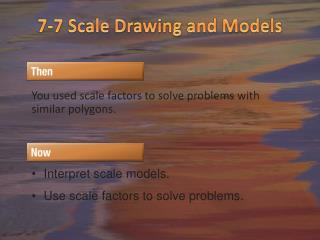DownloadDownload Presentation7-7 Scale Drawing and Models

# 7-7 Scale Drawing and Models

Télécharger la présentation## 7-7 Scale Drawing and Models

- - - - - - - - - - - - - - - - - - - - - - - - - - - E N D - - - - - - - - - - - - - - - - - - - - - - - - - - -
##### Presentation Transcript

1. 7-7 Scale Drawing and Models You used scale factors to solve problems with similar polygons. • Interpret scale models. • Use scale factors to solve problems.

2. Vocabulary Scale model/scale drawing – an object or drawing with lengths proportional to the object it represents. The scale of a model or drawing is the ratio of a length on the model or drawing to the actual length of the object being modeled or drawn.

3. Map Reading The scale on a map relates the size of an object on the map to its actual size. Where do you find the scale on a map? Use the scale and algebra to find the distance between Mishawaka and Kokomo.

4. MAPS The distance between Boston and Chicago on a map is 9 inches. If the scale of the map is 1 inch: 95 miles, what is the actual distance from Boston to Chicago? Method 1 Write and solve a proportion. Let x represent the distance between Boston and Chicago. 1 ● x = 95 ● 9 Cross Products Property x = 855 miles Simplify.

5. Let a = actual distance in miles between Boston and Chicago and m = map distance in inches. Write the scale as , which is 95 miles per inch. So for every inch on the map, the actual distance is 95 miles. Method 2 Write and solve an equation. a = 95 ● m Write an equation. = 95 ● 9 m = 9 = 855 Solve. Answer:The distance between Boston and Chicago is 855 miles. CheckUse dimensional analysis. 

6. MAPSThe distance between Cheyenne, WY, and Tulsa, OK, on a map is 8 inches. If the scale of the map is 1 inch : 90 miles, what is the actual distance from Cheyenne to Tulsa? A. 800 miles B. 900 miles C. 630 miles D. 720 miles

7. Using Scale Factors The scale factor of a drawing or scale model is written as a unit-less ratio in simplest form. Scale factors are always written so that the model length in the ratio comes first.

8. A. SCALE MODEL A miniature replica of a fighter jet is 4 inches long. The actual length of the jet is 12.8 yards. What is the scale of the model? To find the scale, write the ratio of a replica length to an actual length. Answer: The scale of the model is 1 in. : 3.2 yd.

9. B. SCALE MODEL A miniature replica of a fighter jet is 4 inches long. The actual length of the jet is 12.8 yards. How many times as long as the actual is the model jet? To answer this question, find the scale factor of the model. Multiply by a conversion factor that relates inches to yards to obtain a unitless ratio. Answer: The scale factor is 115.2 : 1. That is, the actual jet is 115.2 times as long as the model.

10. A. SCALE MODELA miniature replica of a fire engine is 9 inches long. The actual length of the fire engine is 13.5 yards. What is the scale of the replica? A. 2 in. : 3 yd B. 1 in. : 3 yd C. 2 in. : 5 yd D. 3 in. : 4 yd

11. B. SCALE MODELA miniature replica of a fire engine is 9 inches long. The actual length of the fire engine is 13.5 yards. How many times as long as the model is the actual fire engine? A. 48 B. 54 C. 60 D. 63

12. SCALE DRAWINGGerrard is making a scale model of his classroom on an 11-by-17 inch sheet of paper. If the classroom is 20 feet by 32 feet, choose an appropriate scale for the drawing and determine the drawing’s dimensions. The actual classroom is 20 feet wide. 20 feet ÷ 11 inches = 1.8 feet per inch The actual classroom is 32 feet long. 32 feet ÷ 17 inches = 1.8 feet per inch A scale of 1 inch = 2 feet would be appropriate. So, for every inch on the paper p, let the actual measure a be 2 feet. Write this as an equation. width: a = 2 ● p Write an equation. 20 = 2 ● p a = 20 10 = p Divide each side by 2. length: a = 2 ● p Write an equation. 32 = 2 ● p a = 32 16 = p Divide each side by 2. Answer: 1 in. : 2 ft; 10 in. wide, 16 in. long

13. ARCHITECTUREAlaina is an architect making a scale model of a house in a 15-by-26 inch display. If the house is 84 feet by 144 feet, what would be the dimensions of the model using a scale of 1 in. : 6 ft? A. 14 in. × 24 in. B. 14 in. × 25 in. C. 15 in. × 25 in. D. 15 in. × 26 in.

14. 7-7 Assignment Page 520, 5-12